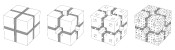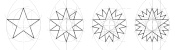mscroggs.co.uk
mscroggs.co.uksubscribe

# Blog

2019-12-08
Just like last year, the year before and the year before, TD and I spent some time in November this year designing a Chalkdust puzzle Christmas card.
The card looks boring at first glance, but contains 9 puzzles. By splitting the answers into two digit numbers, then drawing lines labelled with each number (eg if an answer is 201304, draw the lines labelled 20, 13 and 4), you will reveal a Christmas themed picture. Colouring the regions of the card containing circles red, the regions containing squares green, and the regions containing stars white or yellow will make this picture even nicer.
If you want to try the card yourself, you can download this pdf. Alternatively, you can find the puzzles below and type the answers in the boxes. The answers will be automatically be split into two digit numbers, the lines will be drawn, and the regions will be coloured...

### Similar postsChristmas card 2018Christmas card 2017Christmas card 2016Christmas (2019) is over

Comments in green were written by me. Comments in blue were not written by me.
Thanks for the feedback. (I now understand the need for redaction). My son sent me your link as a Xmas present. I must think of an appropriate retaliation. (What is a PDF?)Think I've fixed 1,6 and 9....8 eludes me, for the moment.
Rob
@Rob: It looks to me like you've made mistakes in questions 1, 6, 8, and 9. The hints from the back of the pdf might help:

1. How many numbers between 1 and 10,000 have 1 as their final digit? How many have 1 as their penultimate digit?

6. How many ways can you write 1? 2? 3? 4? 5? What's the pattern?

8. How many zeros does 10! end in? How many zeros does 20! end in? How many zeros does 30! end in?

9. Carol’s sum is odd. What does this tell you about the 5- and 6-digit numbers?
Matthew
I'm 71, with one good eye left. What am I missing?
1. 400001
2. 1849
3. 2002
4. 130405
5. 120306
6. 53?
7. 171175
8. 59?
9. 313525
Rob

Allowed HTML tags: <br> <a> <small> <b> <i> <s> <sup> <sub> <u> <spoiler> <ul> <ol> <li>
To prove you are not a spam bot, please type "f" then "a" then "c" then "t" then "o" then "r" in the box below (case sensitive):
2018-12-08
Just like last year and the year before, TD and I spent some time in November this year designing a Chalkdust puzzle Christmas card.
The card looks boring at first glance, but contains 10 puzzles. By splitting the answers into pairs of digits, then drawing lines between the dots on the cover for each pair of digits (eg if an answer is 201304, draw a line from dot 20 to dot 13 and another line from dot 13 to dot 4), you will reveal a Christmas themed picture. Colouring the region of the card labelled R red or orange will make this picture even nicer.
If you want to try the card yourself, you can download this pdf. Alternatively, you can find the puzzles below and type the answers in the boxes. The answers will be automatically be split into pairs of digits, lines will be drawn between the pairs, and the red region will be coloured...
If you enjoy these puzzles, then you'll almost certainly enjoy this year's puzzle Advent calendar.

### Similar postsChristmas card 2019Christmas card 2017Christmas card 2016Christmas (2019) is over

Comments in green were written by me. Comments in blue were not written by me.
Someone told me I would like this puzzle and they were right!
Blaine
@Carmel: It's not meant to check your answers. It only shows up red if the number you enter cannot be split into valid pairs (eg the number has an odd number of digits or one of the pairs of digits is greater than 20).
Matthew
The script for checking the answers doesn't work properly
Carmel
Great puzzle problems! Hint on #9: try starting with an analogous problem using smaller numbers (e.g. 3a + 10b). This helped me to see what I had to do more generally.
Noah

Allowed HTML tags: <br> <a> <small> <b> <i> <s> <sup> <sub> <u> <spoiler> <ul> <ol> <li>
To prove you are not a spam bot, please type "sixa-x" backwards in the box below (case sensitive):
2017-12-18
Just like last year, TD and I spent some time in November this year designing a puzzle Christmas card for Chalkdust.
The card looks boring at first glance, but contains 10 puzzles. Converting the answers to base 3, writing them in the boxes on the front, then colouring the 1s black and 2s orange will reveal a Christmassy picture.
If you want to try the card yourself, you can download this pdf. Alternatively, you can find the puzzles below and type the answers in the boxes. The answers will be automatically converted to base 3 and coloured...
 # Answer (base 10) Answer (base 3) 1 0 0 0 0 0 0 0 2 0 0 0 0 0 0 0 3 0 0 0 0 0 0 0 4 0 0 0 0 0 0 0 5 0 0 0 0 0 0 0 6 0 0 0 0 0 0 0 7 0 0 0 0 0 0 0 8 0 0 0 0 0 0 0 9 0 0 0 0 0 0 0 10 0 0 0 0 0 0 0
1. In a book with 116 pages, what do the page numbers of the middle two pages add up to?
2. What is the largest number that cannot be written in the form $$14n+29m$$, where $$n$$ and $$m$$ are non-negative integers?
3. How many factors does the number $$2^6\times3^{12}\times5^2$$ have?
4. How many squares (of any size) are there in a $$15\times14$$ grid of squares?
5. You take a number and make a second number by removing the units digit. The sum of these two numbers is 1103. What was your first number?
6. What is the only three-digit number that is equal to a square number multiplied by the reverse of the same square number? (The reverse cannot start with 0.)
7. What is the largest three-digit number that is equal to a number multiplied by the reverse of the same number? (The reverse cannot start with 0.)
8. What is the mean of the answers to questions 6, 7 and 8?
9. How many numbers are there between 0 and 100,000 that do not contain the digits 0, 1, 2, 3, 4, 5, or 6?
10. What is the lowest common multiple of 52 and 1066?

### Similar postsChristmas card 2016Christmas card 2019Christmas card 2018Christmas (2019) is over

Comments in green were written by me. Comments in blue were not written by me.
@Jose: There is a mistake in your answer: 243 (0100000) is the number of numbers between 10,000 and 100,000 that do not contain the digits 0, 1, 2, 3, 4, 5, or 6.
Matthew
Thanks for the puzzle!
Is it possible that the question 9 is no correct?
I get a penguin with perfect simetrie except at answer 9 : 0100000 that breaks the simetry.
Is it correct or a mistake in my answer?
Thx
Jose
@C: look up something called Frobenius numbers. This problem's equivalent to finding the Frobenius number for 14 and 29.
Lewis
I can solve #2 with code, but is there a tidy maths way to solve it directly?
C

Allowed HTML tags: <br> <a> <small> <b> <i> <s> <sup> <sub> <u> <spoiler> <ul> <ol> <li>
To prove you are not a spam bot, please type "y" then "-" then "a" then "x" then "i" then "s" in the box below (case sensitive):
2016-12-20
Last week, I posted about the Christmas card I designed on the Chalkdust blog.
The card looks boring at first glance, but contains 12 puzzles. Converting the answers to base 3, writing them in the boxes on the front, then colouring the 1s green and 2s red will reveal a Christmassy picture.
If you want to try the card yourself, you can download this pdf. Alternatively, you can find the puzzles below and type the answers in the boxes. The answers will be automatically converted to base 3 and coloured...
 # Answer (base 10) Answer (base 3) 1 0 0 0 0 0 0 0 0 0 2 0 0 0 0 0 0 0 0 0 3 0 0 0 0 0 0 0 0 0 4 0 0 0 0 0 0 0 0 0 5 0 0 0 0 0 0 0 0 0 6 0 0 0 0 0 0 0 0 0 7 0 0 0 0 0 0 0 0 0 8 0 0 0 0 0 0 0 0 0 9 0 0 0 0 0 0 0 0 0 10 0 0 0 0 0 0 0 0 0 11 0 0 0 0 0 0 0 0 0 12 0 0 0 0 0 0 0 0 0
1. The square number larger than 1 whose square root is equal to the sum of its digits.
2. The smallest square number whose factors add up to a different square number.
3. The largest number that cannot be written in the form $$23n+17m$$, where $$n$$ and $$m$$ are positive integers (or 0).
4. Write down a three-digit number whose digits are decreasing. Write down the reverse of this number and find the difference. Add this difference to its reverse. What is the result?
5. The number of numbers between 0 and 10,000,000 that do not contain the digits 0, 1, 2, 3, 4, 5 or 6.
6. The lowest common multiple of 57 and 249.
7. The sum of all the odd numbers between 0 and 66.
8. One less than four times the 40th triangle number.
9. The number of factors of the number $$2^{756}$$×$$3^{12}$$.
10. In a book with 13,204 pages, what do the page numbers of the middle two pages add up to?
11. The number of off-diagonal elements in a 27×27 matrix.
12. The largest number, $$k$$, such that $$27k/(27+k)$$ is an integer.

### Similar postsChristmas card 2017Christmas card 2019Christmas card 2018Christmas (2019) is over

Comments in green were written by me. Comments in blue were not written by me.
@Matthew: Thank you for the prompt response! It makes sense now and perhaps I should have read a little closer!
Dan Whitman
@Dan Whitman: Find the difference between the original number and the reverse of the original. Call this difference $$a$$. Next add $$a$$ to the reverse of $$a$$...
Matthew
In number 4 what are we to take the difference between? Do you mean the difference between the original number and its reverse? If so when you add the difference back to the reverse you simply get the original number, which is ambiguous. I am not sure what you are asking us to do here.
Dan Whitman